Definitions and Theorems

Sunday, February 05, 2012

4:59 PM

 Points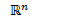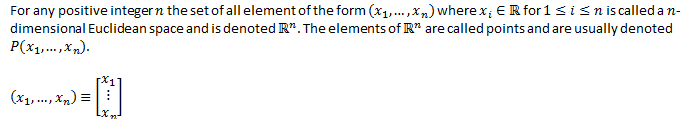Vector addition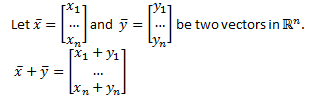Scalar multiplication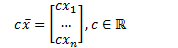Linear combination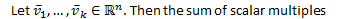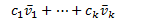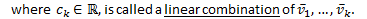Theorem 1.1.1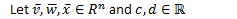1.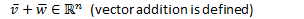1.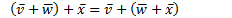2.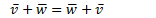1.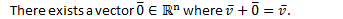1.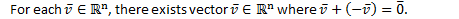1.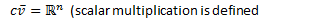1.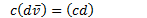1.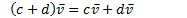2.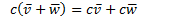1.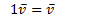Span Spanning set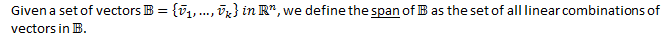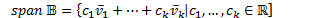Vector Equation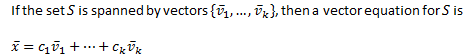Theorem 1.1.2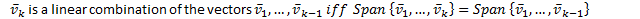Linearly Dependent Linearly Independent Trivial Solution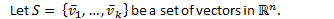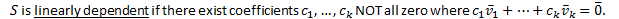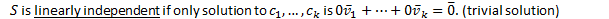Theorem 1.1.3

In order for a spanning set to be as small as possible, it must be linearly independent.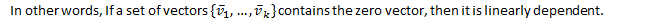Basis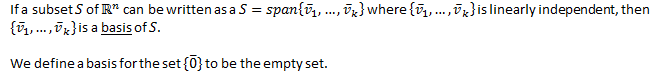Standard Basis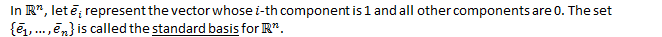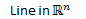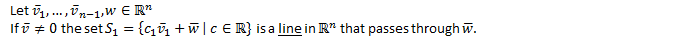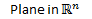K-plane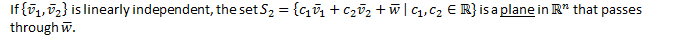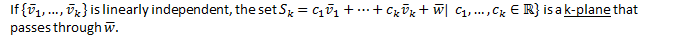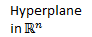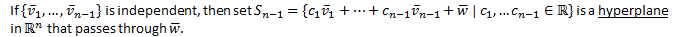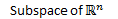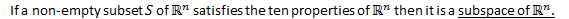Theorem 1.2.1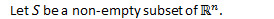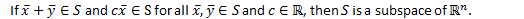(Prove non-empty set, vector addition, and scalar multiplication)

Theorem 1.2.2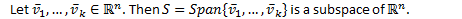Theorem 1.3.1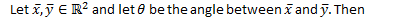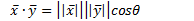Dot product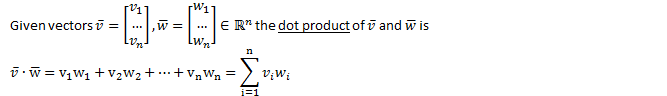Theorem 1.3.2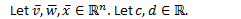1.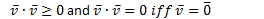1.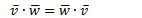1.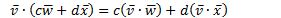Length Norm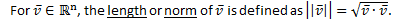Unit Vector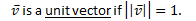Theorem 1.3.3 (Vector Properties)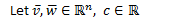1.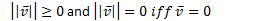1.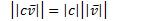1.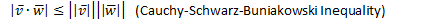1.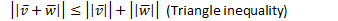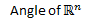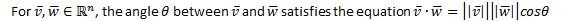Orthogonal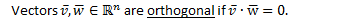Orthogonal set A set of vectors is an orthogonal set if every pair of vectors in the set is orthogonal.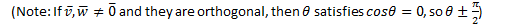Cross Product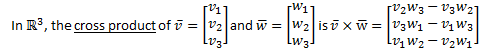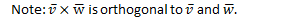Theorem 1.3.4 (Properties of Cross Product)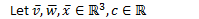1.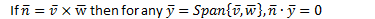1.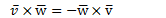1.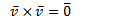1.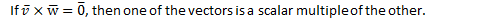1.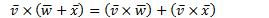1.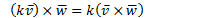1.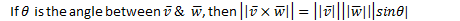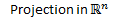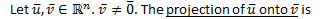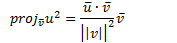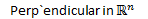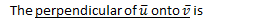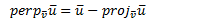Linear equation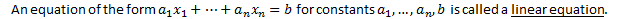Coefficients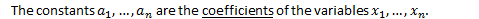System of linear equations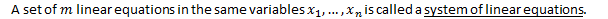(Note: A linear equation can be represented geometrically as a hyperplane.)

 Solution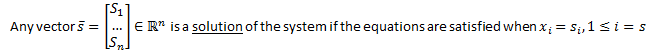Consistent Inconsistent A system of linear equations is consistent if there is at least one solution. Otherwise it is inconsistent.

Theorem 2.1.1

If the following system of linear equations: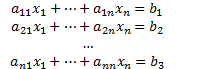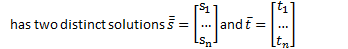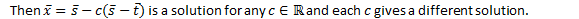(A consistent system with more than one solution has infinitely many solutions.)

 Solution set The set of all solutions of a system of linear equations is the solution set of the system.

 Equivalent If 2 systems of linear equations have the same solution set, then they are equivalent.

 Augmented Matrix For the system of linear equations: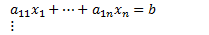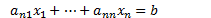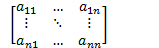Is the coefficient matrix.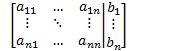Is the augmented matrix.   Note: Rows represent equations and columns represent variables.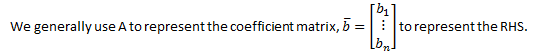Elementary row operations (EROs) There are 3 elementary row operations (EROs) for solving a system of linear equations: Multiply a row by a non-zero scalar. Add a multiple of a row to another row. Swap two rows Row reducing matrix Applying EROs to a matrix is called row reducing a matrix. Row equivalent If there is a sequence of EROs that transform one matrix to another, then the matrices are row equivalent.

Theorem 2.2.1

If augmented matrix are row equivalent, then the corresponding system of linear equations are equivalent.

 Reduced row echelon form (RREF) A matrix is in reduced row echelon form (RREF) if: All rows that have at least non-zero entry are above rows with only zeros. The leftmost non-zero entry in a row is a 1, called a leading one. The leading one in a row is to the right of the leading one in any row above it. Any column containing a leading one has it as its only non-zero entry.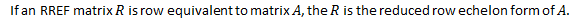Theorem 2.2.2

Every matrix has a unique RREF.

 Free variable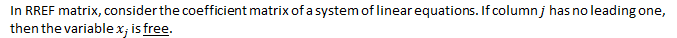Homogeneous system A system of linear equations is homogeneous if the RHS contains only zeros.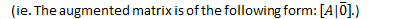Theorem 2.2.3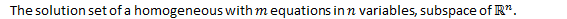Solution space The solution set of a homogeneous system is called the solution space of the system

 Rank The rank of a matrix is the number of leading ones in the RREF of the matrix.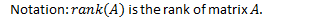Theorem 2.2.4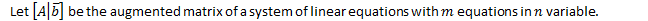1.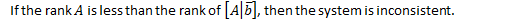2.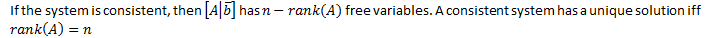1.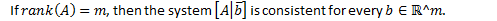Created by Tim Pei with Microsoft OneNote 2010
One place for all your notes and information